Next: 4.3 Results Up: 4.2 Formalism Previous: 4.2.2 Electron-Phonon Coupling

## 4.2.3 Method

In the SCBA the electron self-energy is obtained by summing over all non-crossing diagrams. In this approximation the calculation of the self-energy can be reduced to the following set of equations:(4.18)(4.19)

and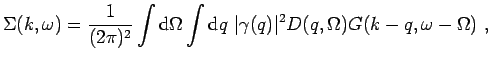(4.20)

where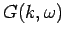andare the free electron and respectively the free phonon Green's functions (see also Fig. 4.1). The frequency integration in Eq. 4.20 can be explicitly completed and one gets(4.21)

where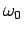is the phonon frequency. Eq. 4.21 is solved self-consistently and the spectral function is determined asIm. The overlap of the polaron state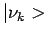(the lowest energy state at a particular momentumwhich in general is an isolated pole in the Green's function) with the free electron state,(4.22)

is called the quasiparticle weight and can be calculated as (25)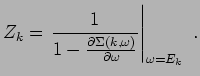(4.23)The neglect of vertex corrections in the SCBA results in a failure of this approximation in the intermediate and strong coupling regime. This is apparent in Fig. 4.2 where a comparison of SCBA and exact QMC results for the H model energy and quasiparticle weight at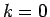is shown. In fact the polaron properties atare better approximated in a regular Rayleigh-Schrodinger (RS) perturbation theory than in SCBA (35). However, at largewhere the polaron energy approaches the phonon frequency, the RS perturbation theory fails and the SCBA which considers configurations involving a large number of phonons provides good results. In order to show that, we compare the SCBA results with the exact ones obtained by using the diagrammatic quantum Monte Carlo technique(36,37) in Fig. 4.3. As can be seen in Fig. 4.3 -a, the dispersion becomes flat when the polaron energy gets close to the the phonon frequency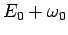,being the bottom of the polaronic band. The flattening is accompanied by a strong reduction of the quasiparticle weight, as shown in Fig. 4.3 -b. The SCBA slightly underestimates the energy corrections in the flat dispersion region but otherwise captures all these features successfully.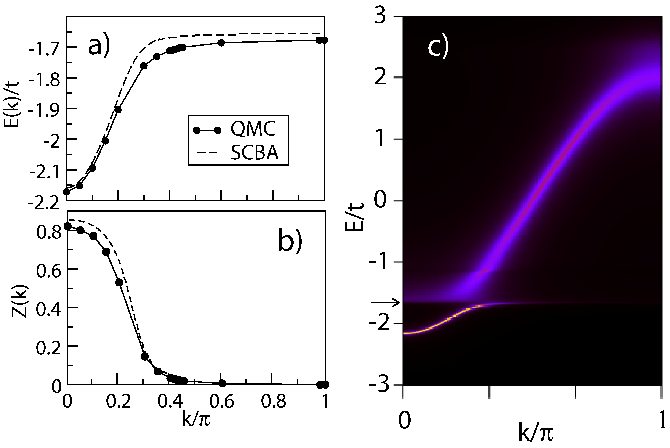The H polaron physics at small coupling has been investigated with different techniques for many years and is rather well understood now. The polaron character changes from almost free electron at the bottom of the band to a one phonon plus one electron state in the flat dispersion region where almost all the momentum is carried by the phonon. The states below the one phonon thresholdare bound states(11), characterized by delta peaks in the spectral representation. Above it, there is a continuum of states and the electron self-energy acquires a finite imaginary part. However, at large energies relative tothe self-energy's imaginary part is small and the spectral representation is characterized by sharp peaks with a dispersion close to the free electron one. In a photoemission experiment the dispersion of the high intensity peak would exhibit something resembling a kink, although a small gap at the phonon energy and sudden onset of broadening appears at the same time. The spectral function at momenta corresponding to energies well below the phonon frequency will consist of a sharp peak followed by a broad satellite, such as it is observed in surface studies of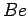(19) for instance. This can be seen in Fig. 4.3 c) where a false color plot of spectral intensity obtained with SCBA is shown (see also Fig. 4.8 -a). The physics discussed in this paragraph (i.e. the dispersion flattening and the gap in the polaron spectrum) is presumably true even for very small electron-phonon couplings. However, for such small couplings these features are too small to be captured experimentally or even numerically.Next: 4.3 Results Up: 4.2 Formalism Previous: 4.2.2 Electron-Phonon Coupling
© Cyrill Slezak